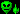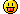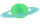Page 1 of 3 [ 37 posts ] Go to page 1, 2, 3  Next
 Print view Previous topic | Next topic
Author Message
Team:Rank: Director
Main: Elryk
Level: 3265

Joined: Sat Oct 15, 2005 12:34 am
Posts: 621
A lot of people may be wondering "How do the best players come up with their setups?" The answer is really quite simple. They calculate possible setups using various formulas. I have come up with a fairly easy way to calculate what your augs and skills will do for your damage, shields, energy and many other stats. Rather than giving you one huge equation to try to solve all at once, I have broken the very long process into 4 smaller, more manageable equations that most people will be able to solve to get the final results of their augs and skills.

Before we start, there are a few things you need to know. First, calculating ship stats using these formulas does require some basic knowledge of algebra, or at least some common sense to figure out what the letters and numbers mean. Second, these formulas don't work on RoF and may not work for Stealth, but have been tested and work perfectly for every other stat. If anyone wants to add to this thread and make the appropriate adjustments to my formulas to figure stealth and rate of fire that would probably help make this a more complete explanation, and I will edit this post to include the new formulas with references and credits going to the person that posts them.

I have listed the variable definitions at the end with a short explanation given by an example. Pay attention to the notations marked by * and ^ in the definitions section. One should also note that fixed values given by items (such as hull expanders) should be figured into the starting or original value before you begin solving these equations.

V1=(S*A1*A2*A3....)
V2=((V1-S)*AT*0.04)+((V1-S)*IT*0.02)
V3=V1+V2
F=V3*M1*M2*(Sk1+1)*(Sk2+1)

Variable definitions:
V1-value before aug tweaking and imperial tweaking
V2-value of aug tweaking and imperial tweaking bonuses
V3-value after aug tweaking and imperial tweaking
S-starting or original value
A1, A2, A3- aug values *
AT-your aug tweaking LEVEL
IT-your imperial tweaking LEVEL
Sk1, Sk2-skills ^
M1, M2-item mods *^
F=final value

*any percentage type values (such as augs, skills or mods) should be put into this formula by dividing by 100 and then adding 1, so a 50% aug, skill or mod would be put in as 1.5
^you can multiply as many skills or mods as necessary here. note that the bonus of skills is simply the level of that skill multiplied by the bonus that skill gives then divided by 100. Support 20 on a freighter would be figured as (20*9)/100=1.8.

For my example, I am going to use a wingship with 2 minor speed augs and a slipstream to calculate speed. I will start by listing the stats of the appropriate gear and ignoring all other stats for this example. We will assume the user of this ship has no skills that modify speed, aug tweaking 5, and no imperial tweaking.

Wingship base speed: 80
Minor Speed Aug bonus: 10%
Slipstream speed bonus: 25%

First lets calculate the ships speed with the 2 minor speed augs (no aug tweaking yet). You will notice i skipped a small step that is listed in the notations after the formulas. Refer to the notations to find out how to make your aug values fit into this formula. I did this to keep the main parts of the calculations clean and easy to understand.
V1 = (S*A1*A2*A3....)
V1 = (80*1.1*1.1) = 96.8
V1 = 96.8
Notice there is a decimal here, thats important, do not round it off yet.

Now lets calculate how much aug tweaking will help.
V2 = ((V1-S)*AT*0.04)+((V1-S)*IT*0.02)
V2 = ((96.8-80)*5*0.04)+((96.8-80)*0*0.02) = 16.8*5*0.04 = 3.36
V2 = 3.36
Notice again that we have a decimal, again, do not round it off yet

Then we add V1 and V2 to get the total speed after we figure for augs and aug tweaking.
V3 = V1+V2
V3 = 96.8+3.36 = 100.16
V3 = 100.16
Once again, we have a decimal, leave it there.

Finally, lets figure out how much speed the slipstream will add and how much your skills will effect the speed. Since the person using this ship has no speed changing skills, we can simply ignore that part of the final step.
F = V3*M1*M2*(Sk1+1)*(Sk2+1)
F = 100.16*1.25 = 125.2
F = 125.2
Now we can round the decimal off and we find that this wingship, used by a person with aug tweaking 5, no imperial tweaking, and no speed modifying skills would go 125.

Hopefully I have made everything clear enough so that you can now start calculating your own ship stats with your own unique setups. Enjoy!Tue Dec 04, 2007 11:49 pmTeam:Rank:
Main: Limpa
Level: 3158

Joined: Mon Jan 16, 2006 11:09 am
Posts: 506
thatch speed 39
6 heph augs. Speed -15% each

V1=39*0.85*0.85*0.85*0.85*0.85*0.85 = 14.708831109375
V2=((14.708831109375-39)*25*0.04)+((14.708831109375-39)*3*0.02) = -25.7486390240625
V3=-11,0398079146875Wed Dec 05, 2007 2:36 amMain: Thummmper
Level: 3066

Joined: Sat Dec 24, 2005 8:19 am
Posts: 0
Please remove this noise.

Last edited by Thummmper on Wed Dec 05, 2007 7:06 pm, edited 1 time in total.

Wed Dec 05, 2007 3:16 amTeam:Rank:
Main: Limpa
Level: 3158

Joined: Mon Jan 16, 2006 11:09 am
Posts: 506
V1=39*0,85*0,85*0,85*0,85*0,85*0,85 = 14,708831109375
V2=((39-14,708831109375)*25*0,04)+((39-14,708831109375)*3*0,02)=25,7486390240625
V3=40,4574701334375Wed Dec 05, 2007 3:54 amJoined: Mon Jun 11, 2007 4:39 pm
Posts: 30
Don't forget to follow the rules of BODMASWed Dec 05, 2007 8:50 amTeam:Rank:
Main: Limpa
Level: 3158

Joined: Mon Jan 16, 2006 11:09 am
Posts: 506
LichG wrote:
Don't forget to follow the rules of BODMASyou show then, how its calculated...

Wed Dec 05, 2007 10:30 amJoined: Mon Jun 11, 2007 4:39 pm
Posts: 30
Ah no screw maths, i just use rough stats, just saying use that if your not.

Wed Dec 05, 2007 10:41 amMain: Jixis
Level: 645

Joined: Sat Jul 28, 2007 5:17 pm
Posts: 0
Location: Cardboard Box, Antarctica
LichG wrote:
Don't forget to follow the rules of BODMASThought it was bedmas .....

_________________
"I feel sorry for people who don't drink, because when they wake up in the morning, that's the best they'll feel all day." --Frank Sinatra

Wed Dec 05, 2007 12:24 pmMain: Churlish
Level: 1171

Joined: Mon Nov 05, 2007 9:35 pm
Posts: 102
2 things:

1, could you add Skills to your example calculation so I can check my working.

2, URJUHH could you tell what your final speed was? and if you have any tweaking, without tweaking i see your speed as 16 using my calculation.

Wed Dec 05, 2007 12:37 pmMain: Goz
Level: 1564

Joined: Sat Dec 17, 2005 9:39 pm
Posts: 83
Location: Eredar US, Gozmatic (Horde)
never mind, I spoke wrongly

_________________
2nd place is just another way to say 1st loser.

Wed Dec 05, 2007 12:43 pmTeam:Rank: Officer
Main: Retyu
Level: 3903

Joined: Wed Feb 23, 2005 7:15 am
Posts: 171
I just look at an aug and say: Damage, cool. Shields Crap. Mad Sci Cool. I think we all know why my DS is crap for PVP.

Wed Dec 05, 2007 2:03 pmMain: Thummmper
Level: 3066

Joined: Sat Dec 24, 2005 8:19 am
Posts: 0
*******************_THIS_LINE_IS_JUST_TO_WIDEN_THE_SCREEN_*************************

ARG! Back to basics. Had to work this from the results. All corrections are welcome.

EDIT: I omitted Skill modifiers... as I have none and didn't think about them when coming up with this. Also made several corrections.

S = Base Speed
VA = Aug modified by AT & IT
FSA = Semi final Speed modified by just the Augs
SM = Speed modified by modded gear
FSM = total of all gear mods
FS = end results

VA1 = 1+(A1*(1+AT*0.04+IT*0.02))
VA2 = 1+(A2*(1+AT*0.04+IT*0.02))
VA3 = 1+(A3*(1+AT*0.04+IT*0.02))
VA4 = 1+(A4*(1+AT*0.04+IT*0.02))
VA5 = 1+(A5*(1+AT*0.04+IT*0.02))
VA6 = 1+(A6*(1+AT*0.04+IT*0.02))
FSA = S*VA1*VA2*VA3*VA4*VA5*VA6
SM1 = 1+M1
SM2 = 1+M2
SM3 = 1+M3
...
FSM = SM1 * SM2 * SM3 * ...
FS = FSA * FSM

For a 6 Heph Thatch with no modded gear equipped:
S = 39
Heph Speed: -15%
AT = 25, IT = 3
VA1 = 1+(A1*(1+AT+IT)) = 1+(-0.15*(1+25*0.04+3*0.02)) = 1-0.15*2.06 = 1-0.309 = 0.691
VA2 = 1+(A2*(1+AT+IT)) = 1+(-0.15*(1+25*0.04+3*0.02)) = 1-0.15*2.06 = 1-0.309 = 0.691
VA3 = 1+(A3*(1+AT+IT)) = 1+(-0.15*(1+25*0.04+3*0.02)) = 1-0.15*2.06 = 1-0.309 = 0.691
VA4 = 1+(A4*(1+AT+IT)) = 1+(-0.15*(1+25*0.04+3*0.02)) = 1-0.15*2.06 = 1-0.309 = 0.691
VA5 = 1+(A5*(1+AT+IT)) = 1+(-0.15*(1+25*0.04+3*0.02)) = 1-0.15*2.06 = 1-0.309 = 0.691
VA6 = 1+(A6*(1+AT+IT)) = 1+(-0.15*(1+25*0.04+3*0.02)) = 1-0.15*2.06 = 1-0.309 = 0.691
FSA = S* VA1* VA2*VA3*VA4*VA5*VA6 = 39*0.691*0.691*0.691*0.691*0.691*0.691 = 4.246
SM1 = 1+M1 = 1
SM2 = 1+M2 = 1
SM3 = 1+M3 = 1
FSM = SM1 * SM2 * SM3 * ... = 1*1*1*... = 1
FS = FSA * FSM = 4.246 * 1 = 4.246 rounded to 4

For a 6 Heph Thatch with no modded gear equipped:
S = 39
Heph Speed: -15%
AT = 23, IT = 3
VA1 = 1+(A1*(1+AT+IT)) = 1+(-0.15*(1+23*0.04+3*0.02)) = 1-0.15*1.98 = 1-0.297 = 0.703
VA2 = 1+(A2*(1+AT+IT)) = 1+(-0.15*(1+23*0.04+3*0.02)) = 1-0.15*1.98 = 1-0.297 = 0.703
VA3 = 1+(A3*(1+AT+IT)) = 1+(-0.15*(1+23*0.04+3*0.02)) = 1-0.15*1.98 = 1-0.297 = 0.703
VA4 = 1+(A4*(1+AT+IT)) = 1+(-0.15*(1+23*0.04+3*0.02)) = 1-0.15*1.98 = 1-0.297 = 0.703
VA5 = 1+(A5*(1+AT+IT)) = 1+(-0.15*(1+23*0.04+3*0.02)) = 1-0.15*1.98 = 1-0.297 = 0.703
VA6 = 1+(A6*(1+AT+IT)) = 1+(-0.15*(1+23*0.04+3*0.02)) = 1-0.15*1.98 = 1-0.297 = 0.703
FSA = S* VA1* VA2*VA3*VA4*VA5*VA6 = 39*0.703*0.703*0.703*0.703*0.703*0.703 = 4.7
SM1 = 1+M1 = 1
SM2 = 1+M2 = 1
SM3 = 1+M3 = 1
FSM = SM1 * SM2 * SM3 * ... = 1*1*1*... = 1
FS = FSA * FSM = 4.7 * 1 = 4.7 rounded to 5

I have AT 23 & IT 3 and my 6 Heph Thatch, with no modded gear equipped, shows a speed of 5.

Last edited by Thummmper on Wed Dec 05, 2007 7:28 pm, edited 4 times in total.

Wed Dec 05, 2007 2:46 pmMain: Churlish
Level: 1171

Joined: Mon Nov 05, 2007 9:35 pm
Posts: 102
This is for Sheilds, Capacity, Speed and Energy right anything else?Wed Dec 05, 2007 5:01 pmMain: Goz
Level: 1564

Joined: Sat Dec 17, 2005 9:39 pm
Posts: 83
Location: Eredar US, Gozmatic (Horde)
NOT for rate of fire/recoil mind you

_________________
2nd place is just another way to say 1st loser.

Wed Dec 05, 2007 5:03 pmMain: Thummmper
Level: 3066

Joined: Sat Dec 24, 2005 8:19 am
Posts: 0
pearsoa wrote:
This is for Sheilds, Capacity, Speed and Energy right anything else?Again I did this just for Speed and it is missing Skill mods as I have no Skills that modify my speed at present... that I know of.

Wed Dec 05, 2007 7:02 pmDisplay posts from previous:  Sort byPage 1 of 3 [ 37 posts ] Go to page 1, 2, 3  Next

#### Who is online

Users browsing this forum: No registered users and 1 guest

 You cannot post new topics in this forumYou cannot reply to topics in this forumYou cannot edit your posts in this forumYou cannot delete your posts in this forumYou cannot post attachments in this forum

 Jump to:  Select a forum ------------------ Development Notes    Testing Center    Updates Archive    News Archive Community    Off Topic Discussion    Star Sonata General Discussion       Ship Submissions       Community Introductions       Client Mods       Art & Avatars       Screenshots & Gameplay Videos Star Sonata    Suggestions       Suggestions Under Consideration          Completed       Rejected Suggestions       Suggestions Voting System       Suggestion Proposals    Team Politics    Outer Rim Outpost Support    Help    Guides    Bugs       Bug Reports: Graphics       Bug Reports: Game play       Bug Reports: UI       Bug Reports: Misc & Website       Bug Archive                Class Rebalance Bugs                Class Rebalance Official ChangelogPowered by phpBB © phpBB Group.